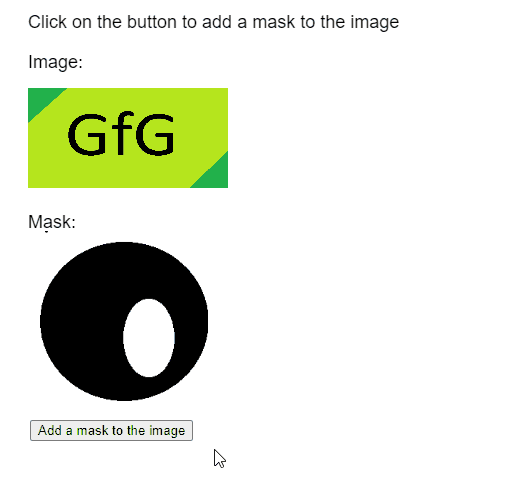GFG App
Open AppBrowser
Continue

The mask() method of p5.Image in p5.js library is used to apply the given mask to the image. This is done by using the alpha channel of the mask image as the alpha channel of this image.

Syntax:

```mask( srcImage )
```

Parameters: This function accepts a single parameter as mentioned above and described below.

• srcImage: It is a p5.Image that would be used as the mask to be applied.

The following libraries are included in the “head” section of the HTML page while implementing the following example.

<script src=”p5.Image.js”></script>
<script src=”p5.min.js”></script>

Example: The example below illustrates the mask() method in p5.js library.

## Javascript

 `function` `preload() { ` `    ``img_orig = ` `      ``loadImage(``"sample-image.png"``); ` `    ``img_mask = ` `      ``loadImage(``"image-mask.png"``); ` `} ` ` `  `function` `setup() { ` `    ``createCanvas(500, 500); ` `    ``textSize(20); ` ` `  `    ``btnBlur = ` `      ``createButton(``"Add a mask to the image"``); ` `    ``btnBlur.position(30, 420); ` `    ``btnBlur.mousePressed(applyMask); ` `} ` ` `  `function` `draw() { ` `    ``clear(); ` ` `  `    ``text(``"Click on the button to add "` `+ ` `         ``"a mask to the image"``, 20, 20); ` `    ``text(``'Image:'``, 20, 60); ` `    ``image(img_orig, 20, 80, 200, 100); ` ` `  `    ``text(``"Mask:"``, 20, 220); ` `    ``image(img_mask, 20, 220, 180, 180); ` `} ` ` `  `function` `applyMask() ` `{ ` `    ``// Apply the given mask to the image ` `    ``img_orig.mask(img_mask); ` `} `

Output:Online editor: https://editor.p5js.org/
Environment Setup: https://www.geeksforgeeks.org/p5-js-soundfile-object-installation-and-methods/